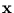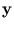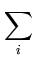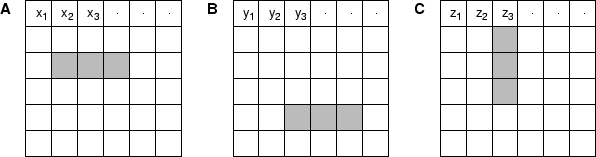Next: 6.2 Methods Up: 6.1 Visual guided grasping Previous: 6.1.1 Related work

## 6.1.2 High-dimensional image data

The visuomotor model associates image data with an arm posture. However, the original images cannot be used as part of a training pattern for a mixture of local PCA. Image processing is required, since the dimensionality of the original images is too high and the correlation between neighboring pixels too low. This problem is illustrated in the following example.

A mixture model is trained on images that show an enlongated object that can be grasped. Figure 6.1 displays sample images, which show position and orientation of the object. For simplicity, the images are binary, and the object occupies three pixels. Each image can be written as a vector. Let, E be the squared distance between two image vectorsand:

 E =(xi - yi)2    . (6.1)

In figure 6.1, the squared distance between A and B is E = 6. The same value holds for all cases in which two images have no overlapping object pixels. These images are in the following called `disjoint images'. On the other hand, the distance between A and C is smaller, namely E = 4. Thus, rotated objects lie closer to each other than objects at different positions. Two problems result from these facts. First, a density model used to describe the distribution of the disjoint images is meaningless if they all have the same distance to each other. Second, in training, the centers of the mixture model would distribute among disjoint images and average over overlapping images (because of the smaller distance in the second case). Thus, the orientation of the object gets lost. Therefore, the images must be preprocessed.Next: 6.2 Methods Up: 6.1 Visual guided grasping Previous: 6.1.1 Related work
Heiko Hoffmann
2005-03-22